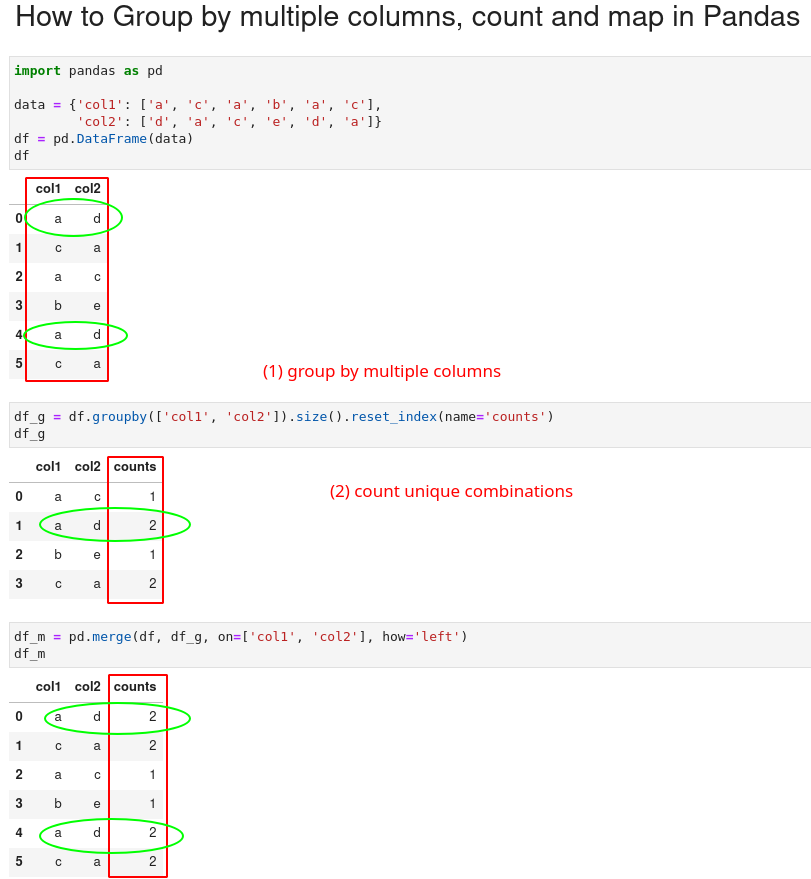To group by two or multiple columns, count unique combinations and map the result we can chain two Pandas methods:

• `groupby()`
• `size()`
``````df.groupby(['col1', 'col2']).size()
``````

The picture below shows all the steps and the final result:Let's create a sample DataFrame and explain all the steps in details:

``````import pandas as pd

data = {'col1': ['a', 'c', 'a', 'b', 'a', 'c'],
'col2': ['d', 'a', 'c', 'e', 'd', 'a']}
df = pd.DataFrame(data)
``````

data looks like:

col1 col2
0 a d
1 c a
2 a c
3 b e
4 a d
5 c a

## Group by two columns and count

To group by multiple columns in Pandas and count the combinations we can chain methods:

``````df_g = df.groupby(['col1', 'col2']).size().reset_index(name='counts')
``````

This gives us a new DataFrame with counts of unique combinations from the columns. We have the original columns plus new columns - `counts` which contains the occurrences:

col1 col2 counts
0 a c 1
1 a d 2
2 b e 1
3 c a 2

## Map count to new column in first DataFrame

Finally we can map the count to the original DataFrame. We will use method `merge` and map on two columns `['col1', 'col2']`:

``````pd.merge(df, df_g, on=['col1', 'col2'])
``````

This gives us:

col1 col2 counts
0 a d 2
1 a d 2
2 c a 2
3 c a 2
4 a c 1
5 b e 1

Or if we like to preserve the order of the original DataFrame we can use left join - `how="left"`:

``````pd.merge(df, df_g, on=['col1', 'col2'], how='left')
``````

How does it work?

• we group by two columns `col1` and `col2`
• the size `method` counts the number of occurrences of each combination
• `reset_index` method reset the index
• rename of the new column to counts and assign counts
• finally map the two DataFrames on multiple columns